## Antiparallels

Notably, the prime five (5) is equal to the sum of the only consecutive primes, 2 + 3. However by the nature it is not going to be as simple as that. Instead they are performing to do a pairing between the Scheme-34 with the Scheme-21.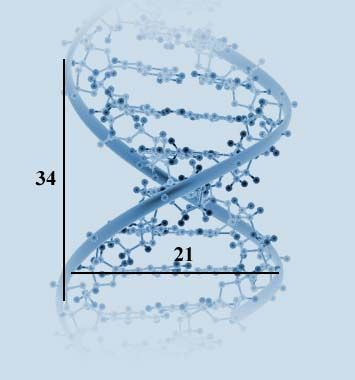By the structure of DNA it is clearly seen that this prime is compromizing the bilateral power of the prime two (2) and grouping power of the prime three (3). Both of these powers turn to the power of the number six (6). This number is the sum and multiplication of three (3) positive numbers in a row 1 + 2 + 3 = 1 x 2 x 3 = 6.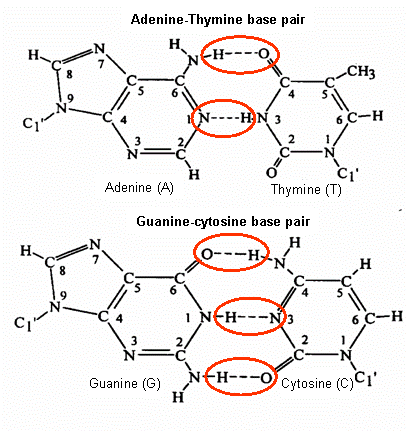So this Scheme-21 is actually coming from 1+2+3+4+5+6 = 21. By observing from many literatures we came to a conclution that it has something to do with a golden ratio (φ) originated by π(π(π(1000th prime)))+1=40 that leads the 168 to 618 primes. So it would be antiparalel directions between π(1000) = 168 to φ = 1000/618 with φ = 1000/618 to π(1000) = 168 (vise versa).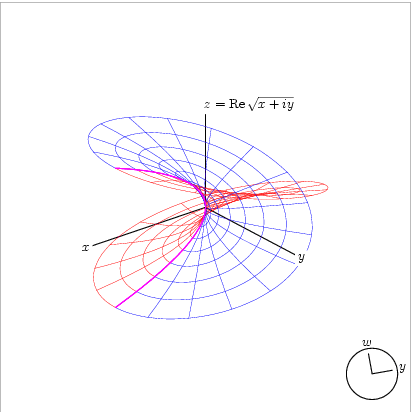By the prime hexagon it will even clearer. Both of the power goes antiparalel in their directions. As the result there would be the period when their instance was created, the period when it was terminated and the period that it shall be raised up.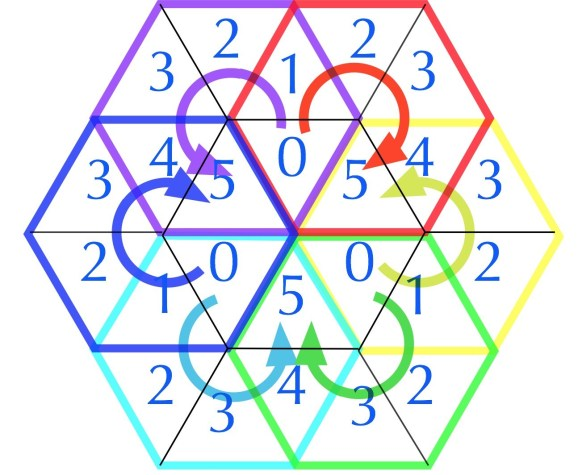Such of this movement goes from 17's and 11's then precissely landed on 5's cell within the hexagon. Each time an instance is raised up then the previous tensor is collected so it would finaly form a configuration of 3,2 → 18+13+12 → 43 as shown below.

``````                             --------------------------------------------------------------
|                                                              |
=======================+====+====+====+====+====+====+====+====+====+=====               |
19 → π(10)            |  2 |  3 |  5 |  7 |  - |  - |  - |  - |  - | 4th  4 x Root      |
-----------------------+----+----+----+----+----+----+----+----+----+-----               |
17 → π(20)            | 11 | 13 | 17 | 19 |  - |  - |  - |  - |  - | 8th  4 x Twin      |
-----------------------+----+----+----+----+----+----+----+----+----+-----               |
13 → π(30) → 12 (Δ1)  | 23 | 29 |  - |  - |  - |  - |  - |  - |  - |10th                |
=======================+====+====+====+====+====+====+====+====+====+===== 1st Twin      |
11 → π(42)            | 31 | 37 | 41 |  - |  - |  - |  - |  - |  - |13th                |
-----------------------+----+----+----+----+----+----+----+----+----+----- 2nd Twin      |
7 → π(60) → 19 (Δ12) | 43 | 47 | 53 | 59 |  - |  - |  - |  - |  - |17th                |
-----------------------+----+----+----+----+----+----+----+----+----+----- 3rd Twin      |
5 → π(72) → 18 (Δ13) | 61 | 67 | 71 |  - |  - |  - |  - |  - |  - |20th ---------------
=======================+====+====+====+====+====+====+====+====+====+===== 4th Twin
3,2 → 18+13+12 → 43  | 73 | 79 | 83 | 89 | 97 | 101| 103| 107| 109|29th
=======================+====+====+====+====+====+====+====+====+====+=====
``````

By this 43 the scheme goes to the limit of π(10) = (2,3.5,7) resulting 2 times prime levels from 10th primes of 29 to 29th prime of 109. See that they are touching the power of prime two (2) by carrying nine (9) cells of prime powers.

In the matrix pictured below, we list the first 24 elements of our domain, take their squares, calculate the modulo 90 congruence and digital roots of each square, and display the digital root factorization dyad for each square and map their collective bilateral 9 sum symmetry (Primesdemystified)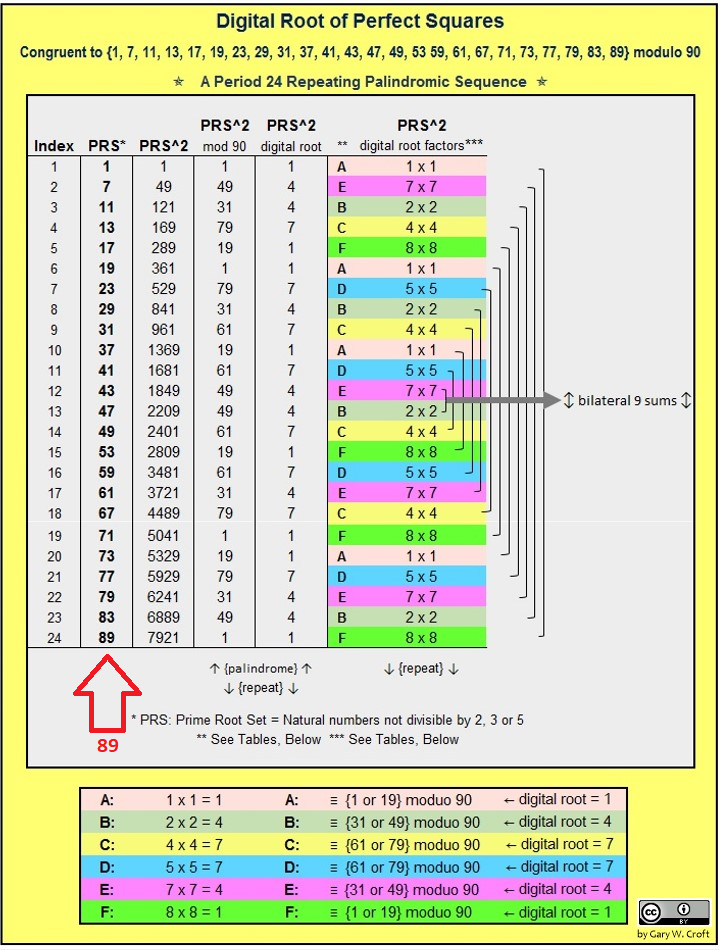Since the prime two (2) has a bigger power of all primes combined then it will force back those power precisely as shown on the tabulation above. So from the movement of the form of (7,5,3,2) it goes to (2,3,5,7) as a new replication of π(10).

``````-----------------------+----+----+----+----+----+----+----+----+----+-----
True Prime Pairs Δ    |  1 |  2 |  3 |  4 |  5 |  6 |  7 |  8 |  9 | Sum
=======================+====+====+====+====+====+====+====+====+====+=====
19 → π(10)            |  2 |  3 |  5 |  7 |  - |  - |  - |  - |  - | 4th  4 x Root
-----------------------+----+----+----+----+----+----+----+----+----+-----
17 → π(20)            | 11 | 13 | 17 | 19 |  - |  - |  - |  - |  - | 8th  4 x Twin
-----------------------+----+----+----+----+----+----+----+----+----+-----
13 → π(30) → 12 (Δ1)  | 23 | 29 |  - |  - |  - |  - |  - |  - |  - |10th ←------------ 10
=======================+====+====+====+====+====+====+====+====+====+===== 1st Twin
11 → π(42)            | 31 | 37 | 41 |  - |  - |  - |  - |  - |  - |13th
-----------------------+----+----+----+----+----+----+----+----+----+----- 2nd Twin
7 → π(60) → 19 (Δ12) | 43 | 47 | 53 | 59 |  - |  - |  - |  - |  - |17th
-----------------------+----+----+----+----+----+----+----+----+----+----- 3rd Twin
5 → π(72) → 18 (Δ13) | 61 | 67 | 71 |  - |  - |  - |  - |  - |  - |20th ←------------ 20
=======================+====+====+====+====+====+====+====+====+====+===== 4th Twin
3 → 18+13+12 → 43    | 73 | 79 | 83 | 89 | 97 | 101| 103| 107| 109|29th ------------→ 30
2 -------------------+----+----+----+----+----+----+----+----+----+-----  ←-- bilateral 9 sums
3 → 18+13+12 → 43    | 73 | 79 | 83 | 89 | 97 | 101| 103| 107| 109|29th ------------→ 30
=======================+====+====+====+====+====+====+====+====+====+===== 4th Twin
5 → π(72) → 18 (Δ13) | 61 | 67 | 71 |  - |  - |  - |  - |  - |  - |20th ←------------ 20
-----------------------+----+----+----+----+----+----+----+----+----+----- 3rd Twin
7 → π(60) → 19 (Δ12) | 43 | 47 | 53 | 59 |  - |  - |  - |  - |  - |17th
``````

This replication is the one that forming 5' vs 3' with 3' vs 5' ends of DNA. One thing we should notify here is that this bilateral movements is originated by the 17's of 100 objects. So the period will cover antiparales of 2 times 100+43 or 286 objects.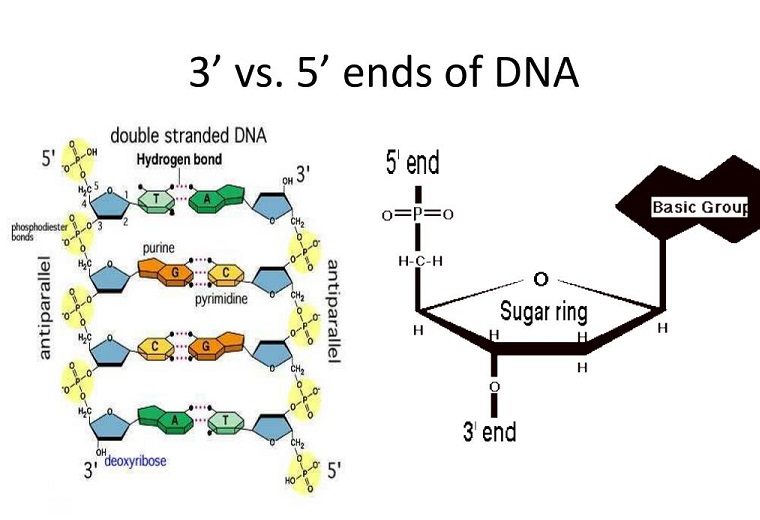In order to facilitate this behaviour the flows within the three (3) inside containers shall have an entrance to the period when the instance was created, the period when it was terminated and the period that it shall be raised up to exchange its existence.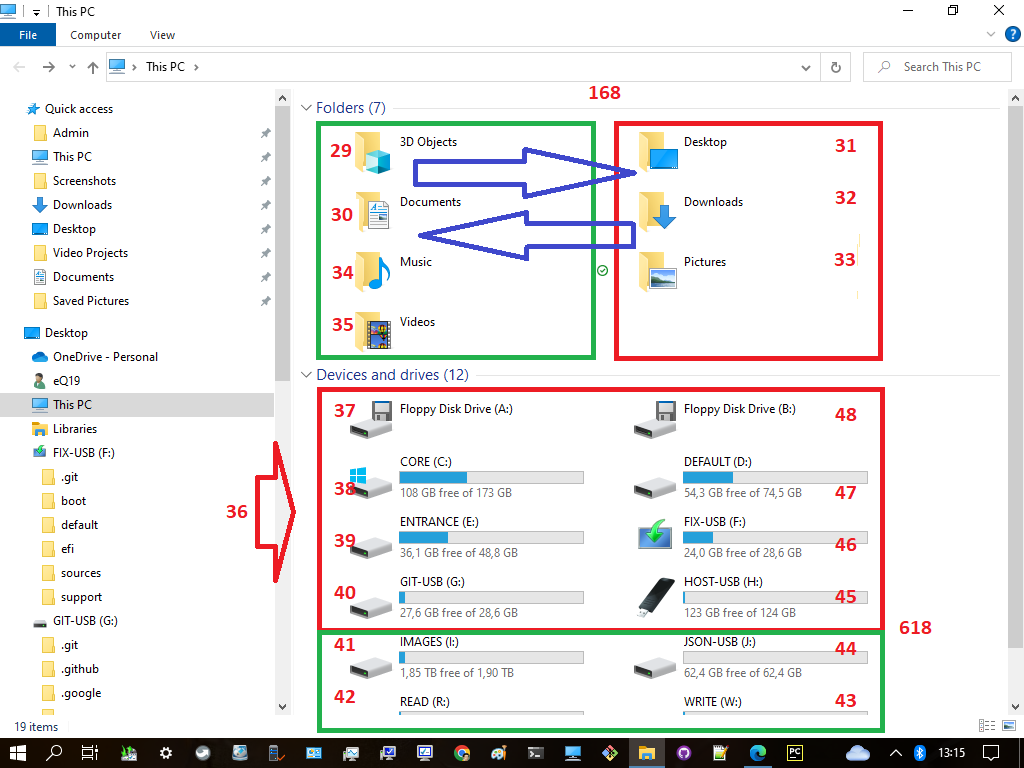The end of this formation is at fifty (50) which at the next level will carry out the regeneration process to 102 via the number one hundred (100) for its correlation with the number two (2).With the help of a computer, we appear these two objects only those related to the intended case. Where we can do mapping or iteration with the data we prepare so that results are obtained more quickly and accurately.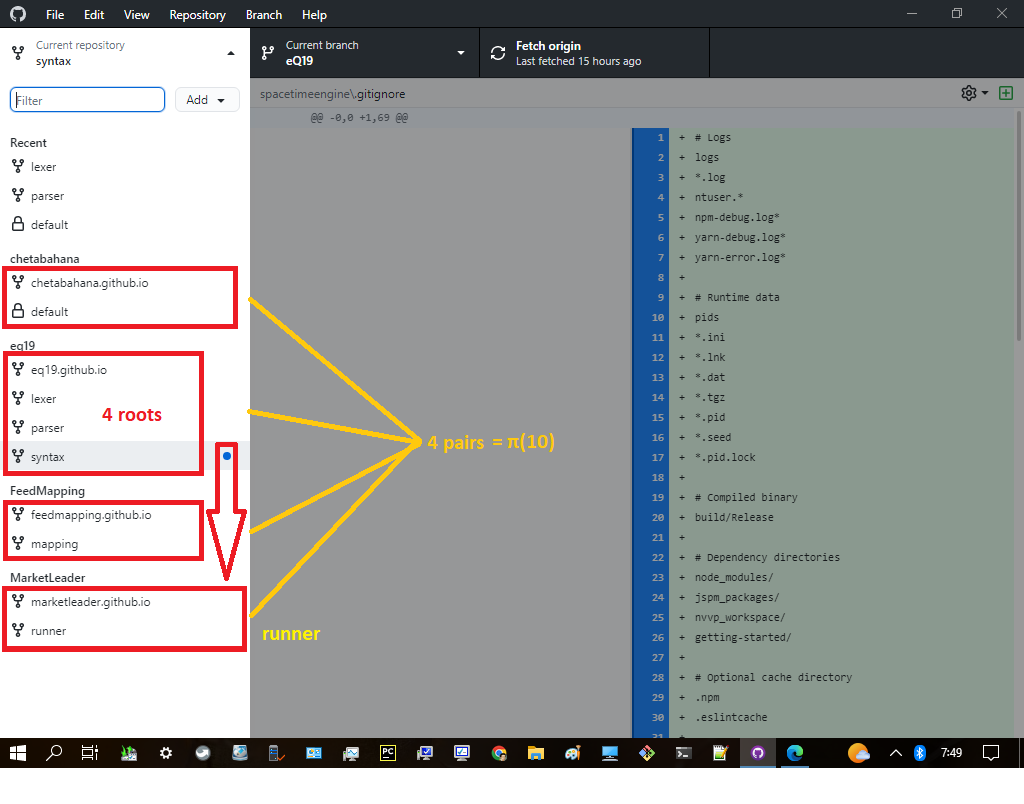For the series of consecutive powers of pi, it has been found that there is no two fall within the same six-cell hexagon. As a matter of a fact it was computed this for pi^32, which has less than a 1/400 chance of occurring randomly.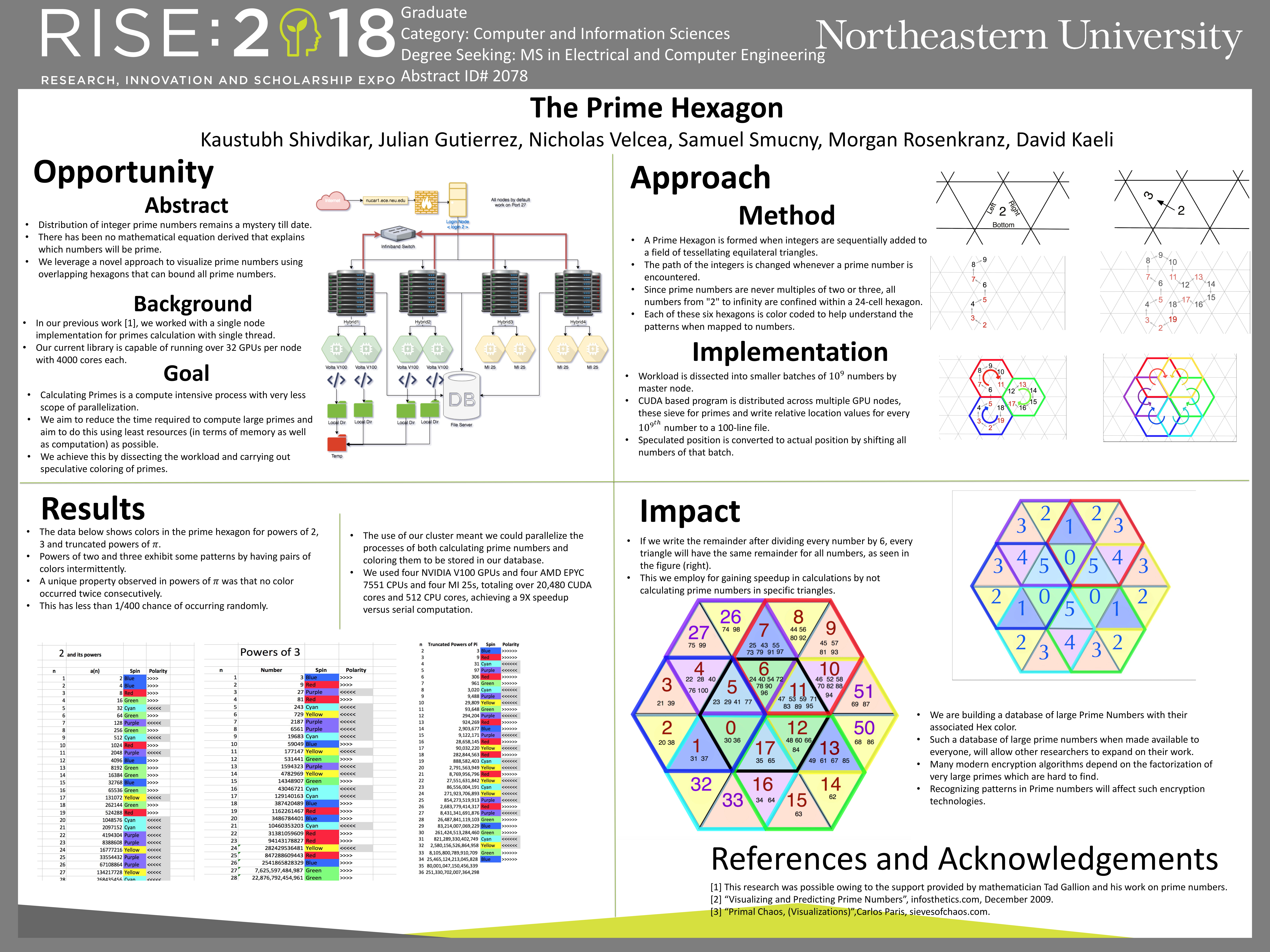This is a kind of connections to display the above complex relation between the individual objects and their tensors. Same case as we have expressed before we will bring further every details particulary on this scope in to another website separately.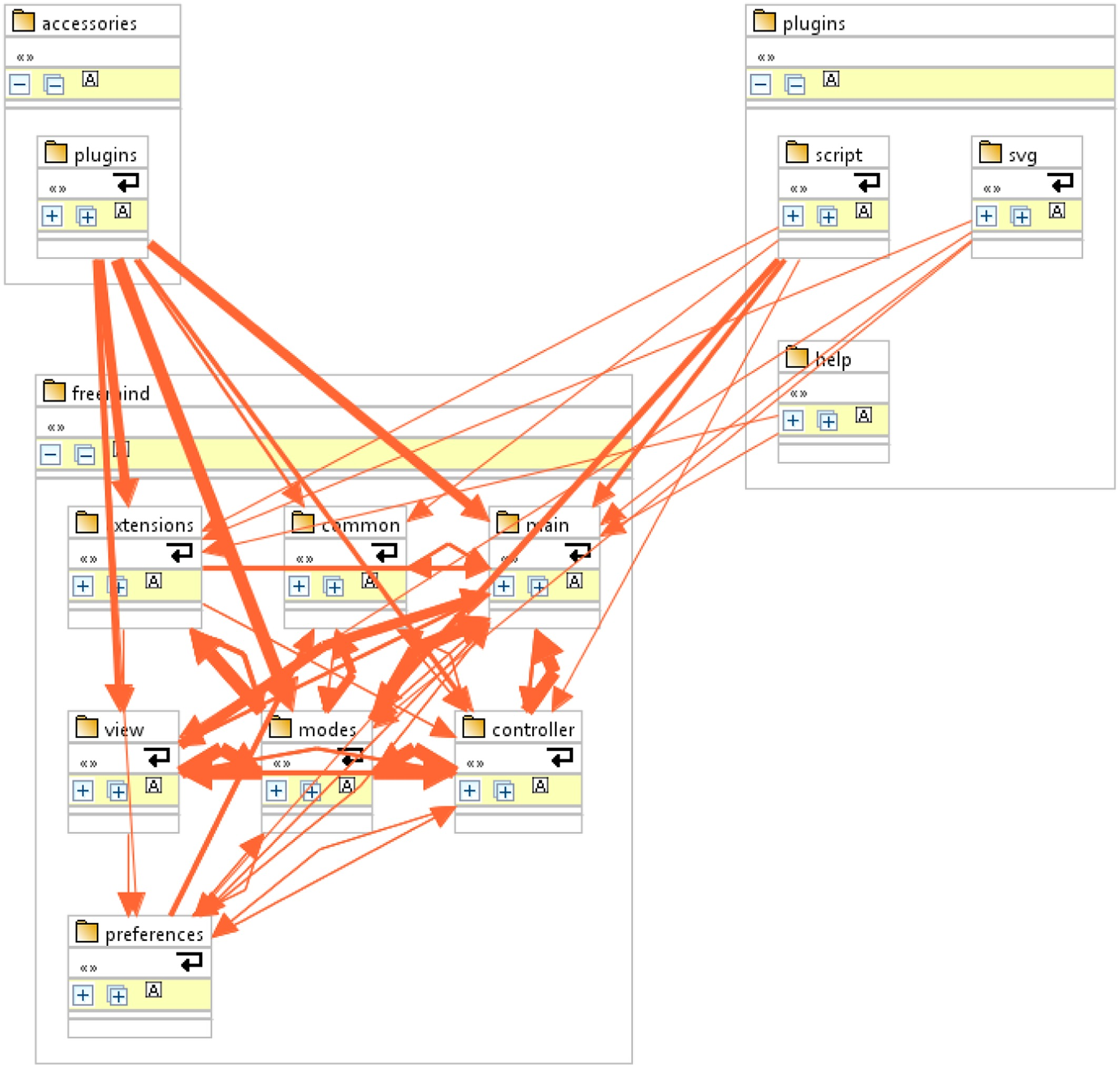By using this method of interpolation between the object and their tensors we finaly found that the Scheme-35 is actualy come from anti paralel behaviour of Scheme-53 which is containing three (3) prime pairs.